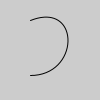# bezier_vertex()#

Specifies vertex coordinates for Bezier curves.

## Examples#def setup():
py5.no_fill()
py5.begin_shape()
py5.vertex(30, 20)
py5.bezier_vertex(80, 0, 80, 75, 30, 75)
py5.end_shape()def setup():
py5.begin_shape()
py5.vertex(30, 20)
py5.bezier_vertex(80, 0, 80, 75, 30, 75)
py5.bezier_vertex(50, 80, 60, 25, 30, 20)
py5.end_shape()


## Description#

Specifies vertex coordinates for Bezier curves. Each call to bezier_vertex() defines the position of two control points and one anchor point of a Bezier curve, adding a new segment to a line or shape. The first time bezier_vertex() is used within a begin_shape() call, it must be prefaced with a call to vertex() to set the first anchor point. This function must be used between begin_shape() and end_shape() and only when there is no MODE parameter specified to begin_shape(). Using the 3D version requires rendering with P3D.

Underlying Processing method: bezierVertex

## Signatures#

bezier_vertex(
x2: float,  # the x-coordinate of the 1st control point
y2: float,  # the y-coordinate of the 1st control point
x3: float,  # the x-coordinate of the 2nd control point
y3: float,  # the y-coordinate of the 2nd control point
x4: float,  # the x-coordinate of the anchor point
y4: float,  # the y-coordinate of the anchor point
/,
) -> None

bezier_vertex(
x2: float,  # the x-coordinate of the 1st control point
y2: float,  # the y-coordinate of the 1st control point
z2: float,  # the z-coordinate of the 1st control point
x3: float,  # the x-coordinate of the 2nd control point
y3: float,  # the y-coordinate of the 2nd control point
z3: float,  # the z-coordinate of the 2nd control point
x4: float,  # the x-coordinate of the anchor point
y4: float,  # the y-coordinate of the anchor point
z4: float,  # the z-coordinate of the anchor point
/,
) -> None


Updated on March 06, 2023 02:49:26am UTC